# Matplotlib - Transformations Tutorial¶

Like any graphics packages, Matplotlib is built on top of a transformation framework to easily move between coordinate systems, the userland data coordinate system, the axes coordinate system, the figure coordinate system, and the display coordinate system.

In 95% of your plotting, you won't need to think about this, as it happens under the hood, but as you push the limits of custom figure generation, it helps to have an understanding of these objects so you can reuse the existing transformations Matplotlib makes available to you, or create your own.

The table below summarizes some useful coordinate systems, the transformation object you should use to work in that coordinate system, and the description of that system. In the Transformation Object column, ax is an Axes instance, and fig is a Figure instance.

### Data Co-ordinates¶

In :
import numpy as np
import matplotlib.pyplot as plt
import matplotlib.patches as mpatches

x = np.arange(0, 10, 0.005)
y = np.exp(-x/2.) * np.sin(2*np.pi*x)

fig, ax = plt.subplots()
ax.plot(x, y)
ax.set_xlim(0, 10)
ax.set_ylim(-1, 1)

plt.show()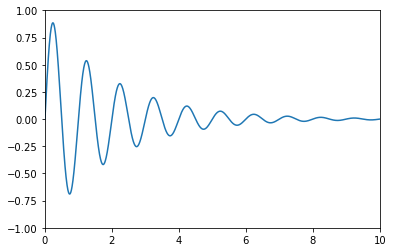• "data" ax.transData The coordinate system for the data, controlled by xlim and ylim.
• "axes" ax.transAxes The coordinate system of the Axes; (0, 0) is bottom left of the axes, and (1, 1) is top right of the axes.
• "figure" fig.transFigure The coordinate system of the Figure; (0, 0) is bottom left of the figure, and (1, 1) is top right of the figure.
• "figure-inches" fig.dpi_scale_trans The coordinate system of the Figure in inches; (0, 0) is bottom left of the figure, and (width, height) is the top right of the figure in inches.
• "display" None, or IdentityTransform() The pixel coordinate system of the display window; (0, 0) is bottom left of the window, and (width, height) is top right of the display window in pixels.
• "xaxis", "yaxis" ax.get_xaxis_transform(), ax.get_yaxis_transform() Blended coordinate systems; use data coordinates on one of the axis and axes coordinates on the other.
In :
x = np.arange(0, 10, 0.005)
y = np.exp(-x/2.) * np.sin(2*np.pi*x)

fig, ax = plt.subplots()
ax.plot(x, y)
ax.set_xlim(0, 10)
ax.set_ylim(-1, 1)

xdata, ydata = 5, 0
xdisplay, ydisplay = ax.transData.transform_point((xdata, ydata))

bbox = dict(boxstyle="round", fc="0.8")
arrowprops = dict(
arrowstyle="->",

offset = 72
ax.annotate('data = (%.1f, %.1f)' % (xdata, ydata),
(xdata, ydata), xytext=(-2*offset, offset), textcoords='offset points',
bbox=bbox, arrowprops=arrowprops)

disp = ax.annotate('display = (%.1f, %.1f)' % (xdisplay, ydisplay),
(xdisplay, ydisplay), xytext=(0.5*offset, -offset),
xycoords='figure pixels',
textcoords='offset points',
bbox=bbox, arrowprops=arrowprops)

plt.show()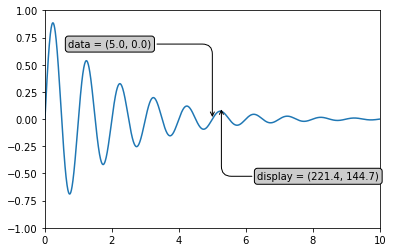### Axes Co-ordinates¶

After the data coordinate system, axes are probably the second most useful coordinate system.

Here the point (0, 0) is the bottom left of your axes or subplot, (0.5, 0.5) is the center, and (1.0, 1.0) is the top right. You can also refer to points outside the range, so (-0.1, 1.1) is to the left and above your axes. This coordinate system is extremely useful when placing text in your axes, because you often want a text bubble in a fixed, location, e.g., the upper left of the axes pane, and have that location remain fixed when you pan or zoom. Here is a simple example that creates four panels and labels them 'A', 'B', 'C', 'D' as you often see in journals.

In :
fig = plt.figure()
for i, label in enumerate(('A', 'B', 'C', 'D')):
ax.text(0.05, 0.95, label, transform=ax.transAxes,
fontsize=16, fontweight='bold', va='top')

plt.show()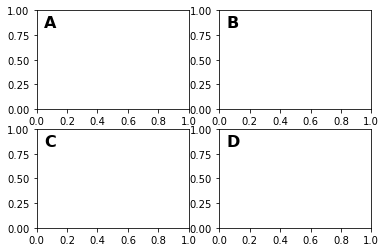In :
fig, ax = plt.subplots()
x, y = 10*np.random.rand(2, 1000)
ax.plot(x, y, 'go', alpha=0.2)  # plot some data in data coordinates

circ = mpatches.Circle((0.5, 0.5), 0.25, transform=ax.transAxes,
facecolor='blue', alpha=0.75)
plt.show()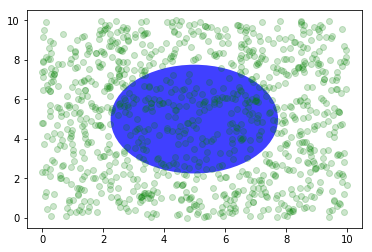### Blended Transformations¶

Drawing in blended coordinate spaces that mix axes with data coordinates are extremely useful, for example, to create a horizontal span which highlights some region of the y-data but spans across the x-axis regardless of the data limits, pan or zoom level, etc. In fact, these blended lines and spans are so useful, we have built-in functions to make them easy to plot (see axhline(), axvline(), axhspan(), axvspan()) but for didactic purposes, we will implement the horizontal span here using a blended transformation. This trick only works for separable transformations, like you see in normal Cartesian coordinate systems, but not on inseparable transformations like the PolarTransform.

In :
import matplotlib.transforms as transforms

fig, ax = plt.subplots()
x = np.random.randn(1000)

ax.hist(x, 30)
ax.set_title(r'$\sigma=1 \/ \dots \/ \sigma=2$', fontsize=16)

# the x coords of this transformation are data, and the
# y coord are axes
trans = transforms.blended_transform_factory(
ax.transData, ax.transAxes)

# highlight the 1..2 stddev region with a span.
# We want x to be in data coordinates and y to
# span from 0..1 in axes coords
rect = mpatches.Rectangle((1, 0), width=1, height=1,
transform=trans, color='yellow',
alpha=0.5)

plt.show()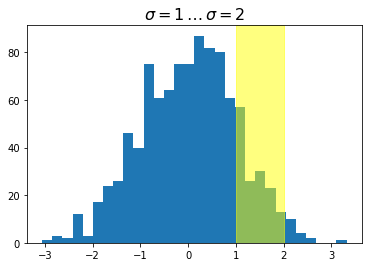**Note:**
The blended transformations where x is in data coords and y in axes coordinates is so useful that we have helper methods to return the versions mpl uses internally for drawing ticks, tick labels, etc.

The methods are matplotlib.axes.Axes.get_xaxis_transform() and matplotlib.axes.Axes.get_yaxis_transform(). So in the example above, the call to blended_transform_factory() can be replaced by get_xaxis_transform:
**trans = ax.get_xaxis_transform()**

### Plotting In Physical Units¶

Sometimes we want an object to be a certain physical size on the plot. Here we draw the same circle as above but in physical units. If done interactively, you can see that changing the size of the figure does not change the offset of the circle from the lower-left corner, does not change its size, and the circle remains a circle regardless of the aspect ratio of the axes.

In :
fig, ax = plt.subplots(figsize=(5, 4))
x, y = 10*np.random.rand(2, 1000)
ax.plot(x, y*10., 'go', alpha=0.2)  # plot some data in data coordinates
# add a circle in fixed-units
circ = mpatches.Circle((2.5, 2), 1.0, transform=fig.dpi_scale_trans,
facecolor='yellow', alpha=0.75)
plt.show()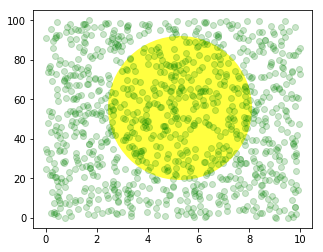If we change the figure size, the circle does not change its absolute position and is cropped.

In :
fig, ax = plt.subplots(figsize=(7, 2))
x, y = 10*np.random.rand(2, 1000)
ax.plot(x, y*10., 'go', alpha=0.2)  # plot some data in data coordinates
# add a circle in fixed-units
circ = mpatches.Circle((2.5, 2), 1.0, transform=fig.dpi_scale_trans,
facecolor='yellow', alpha=0.75)
plt.show()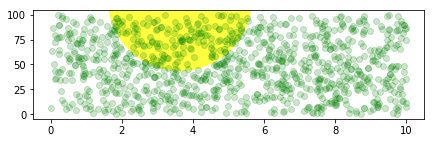In :
fig, ax = plt.subplots()
xdata, ydata = (0.2, 0.7), (0.5, 0.5)
ax.plot(xdata, ydata, "o")
ax.set_xlim((0, 1))

trans = (fig.dpi_scale_trans +
transforms.ScaledTranslation(xdata, ydata, ax.transData))

# plot an ellipse around the point that is 150 x 130 points in diameter...
circle = mpatches.Ellipse((0, 0), 150/72, 130/72, angle=40,
fill=None, transform=trans)
plt.show()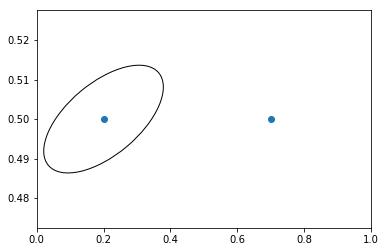Note: The order of transformation matters.

Here the ellipse is given the right dimensions in display space first and then moved in data space to the correct spot. If we had done the **ScaledTranslation** first, then **xdata** and **ydata** would first be transformed to display coordinates **([ 358.4 475.2]** on a 200-dpi monitor) and then those coordinates would be scaled by fig.dpi_scale_trans pushing the center of the ellipse well off the screen (i.e. [ 71680. 95040.]).

### Using Offset Transforms To Create A Shadow Effect¶

Another use of ScaledTranslation is to create a new transformation that is offset from another transformation, e.g., to place one object shifted a bit relative to another object.

Typically you want the shift to be in some physical dimension, like points or inches rather than in data coordinates, so that the shift effect is constant at different zoom levels and dpi settings.

In :
fig, ax = plt.subplots()

# make a simple sine wave
x = np.arange(0., 2., 0.01)
y = np.sin(2*np.pi*x)
line, = ax.plot(x, y, lw=3, color='blue')

# shift the object over 2 points, and down 2 points
dx, dy = 2/72., -2/72.
offset = transforms.ScaledTranslation(dx, dy, fig.dpi_scale_trans)

# now plot the same data with our offset transform;
# use the zorder to make sure we are below the line
ax.plot(x, y, lw=3, color='gray',
zorder=0.5*line.get_zorder())

ax.set_title('Creating a Shadow Effect with an Offset Transform')
plt.show()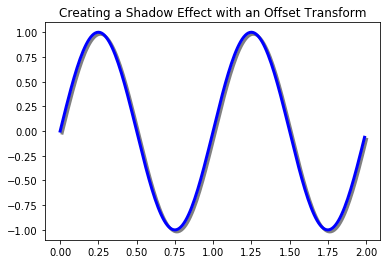**Note** The dpi and inches offset is a common-enough use case that we have a special helper function to create it in matplotlib.transforms.offset_copy(), which returns a new transform with an added offset. So above we could have done:
shadow_transform = transforms.offset_copy(ax.transData, fig=fig, dx, dy, units='inches')

</div>

### The Transformation Pipeline¶

• The ax.transData transform we have been working within this tutorial is a composite of three different transformations that comprise the transformation pipeline from data -> display coordinates.

• Michael Droettboom implemented the transformations framework, taking care to provide a clean API that segregated the nonlinear projections and scales that happen in polar and logarithmic plots, from the linear affine transformations that happen when you pan and zoom.

• There is an efficiency here because you can pan and zoom in your axes which affects the affine transformation, but you may not need to compute the potentially expensive nonlinear scales or projections on simple navigation events.

• It is also possible to multiply affine transformation matrices together, and then apply them to coordinates in one step. This is not true of all possible transformations.

self.transData = self.transScale + (self.transLimits + self.transAxes)

transProjection handles the projection from the space, e.g., latitude and longitude for map data, or radius and theta for polar data, to a separable Cartesian coordinate system. There are several projection examples in the matplotlib. projections package and the best way to learn more is to open the source for those packages and see how to make your own since Matplotlib supports extensible axes and projections.Dolly Solanki